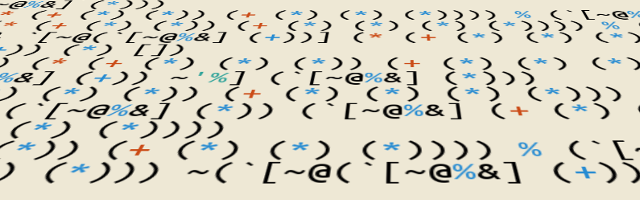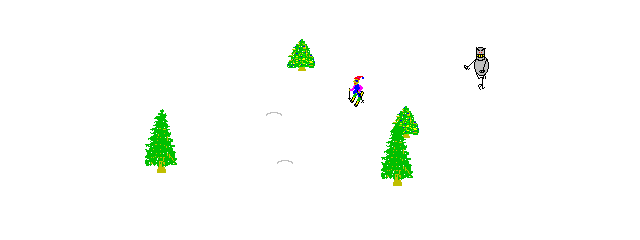# Swearjure is Turing Complete

postedSwearjure development stagnated since I finished Hello Swearjure (For a refresher on Swearjure, see my Swearjure blog post). I blame this on the assumption that it was impossible to have functions containing functions inside it:

``````#(#())
;; IllegalStateException Nested #()s are not allowed
``````

This is, of course, sensible from a language standpoint, but a tragic problem in Swearjure that adds a lot of incidental complexity if we want to do something useful. Remember from the old SICP days, where you learned that

``````(let ((a (+ 1 (* x y)))
(b (- 1 y)))
(+ a b))
``````

and

``````((lambda (a b)
(+ a b))
(+ 1 (* x y))
(- 1 y))
``````

are the same things. It would be really nice if we could utilise this in Swearjure too!

## -»

During Autumn 2014, a friend of Gary Fredericks discovered a new trick. You can actually create functions containing functions, albeit with limited value:

``````(require '[clojure.walk :refer [macroexpand-all]])
#_=> nil
(macroexpand-all '(->> #(+ % %) #(* % %)))
#_=> (fn* [p1__845#] (* p1__845# p1__845#)
(fn* [p1__844#] (+ p1__844# p1__844#)))
``````

If you wonder, `fn*` is just the same as `fn`, except that all the sugar `fn` allows you to do is not allowed. That’s more or less destructuring within the parameter vector, pre- and post conditions, plus some form validation.

What we get back doesn’t seem to make sense, at least not at first sight. Therefore, let’s have look at what happens here, step by step. First, what does `#(+ % %)` really return? One way to check this is by quoting the result, like so:

``````'#(+ % %)
#_=> (fn* [p1__848#] (+ p1__848# p1__848#))

'#()
#_=> (fn* [] ())
``````

So function literals in Clojure are actually expanded before they are evaluated! With that knowledge, it makes a bit more sense why `->>` injects the first function into the other. They both treated as lists, and as specified by the docstring:

``````clojure.core/->>
([x & forms])
Macro
Threads the expr through the forms. Inserts x as the last item
in the first form, making a list of it if it is not a list
already. If there are more forms, inserts the first form as
the last item in second form, etc.
``````

As an example, this is what the `->>` macro does to forms before they are being evaluated:

``````(macroexpand-all '(->> (a b c) (d e f)))
#_=> (d e f (a b c))
``````

And that’s what happens with the `fn*` lists too.

Of course, the question that should pop up for any seasoned Swearjure developer would be: Can we do something to make use of this? The answer depends on whether we can refer to a variable from the outermost function in the innermost one. We can’t do that by using `%`, `%2` and friends, or `%&`. They always make gensyms, which by definition should be a unique symbol not used by anything else:

``````'(->> #(+ % %) #(* % %))
#_=> (->> (fn* [p1__860#] (+ p1__860# p1__860#))
(fn* [p1__861#] (* p1__861# p1__861#)))
``````

As we can see, `#(+ % %)` replaced `%` with `p1__860#`, and `#(+ % %)` replaced `%` with `p1__861#`. So we can create those let bindings as we can in Scheme, but we can’t refer to the variables they point to. Close, but no cigar.

## ->

It occurred to me that we can do the same trick with `->` as well.

``````(macroexpand-all '(-> #() #(+ % %)))
#_=> (fn* (fn* [] ()) [p1__867#]
(+ p1__867# p1__867#))
``````

… Except this doesn’t make any sense: We’ve now replaced the binding form with a form that evaluates to a function. Not exactly what we had in mind.

However, this means that we can replace the binding form! Indeed, this works fine: (I’ve decided to use normal letters to make it easier to read, this stuff is confusing enough as is.)

``````(macroexpand-all '(-> [a] #()))
#_=> (fn* [a] [] ())

(macroexpand-all '(-> [a] #(+ a a)))
#_=> (fn* [a] [] (+ a a))

((-> [a] #(+ a a)) 10)
#_=> 20
``````

You can see that the original, empty binding form is pushed one argument back. This isn’t a problem, it only means it will be part of the function body and will be evaluated. As there is nothing dangerous to evaluate in it – it’s only the empty vector – this isn’t a problem.

Before going on, let’s derail slightly for a short moment: This result in and by itself is really valuable for general Swearjure development. Making functions with multiple arguments was a real mess and hard to work with, so being able to make it easier is really valuable in general.

In addition, we can now define recursive anonymous functions! For example, we can implement factorial:

``````(macroexpand-all '(-> ! #(if (zero? %) 1 (* % (! (- % 1))))))
#_=> (fn* ! [p1__881#]
(if (zero? p1__881#) 1
(* p1__881# (! (- p1__881# 1)))))

((-> ! #(if (zero? %) 1 (* % (! (- % 1))))) 10)
#_=> 3628800
``````

With this technique, it’s actually possible to make a fully Swearjurised version of factorial using only one function literal. This is left as an exercise for the reader.

Now back to the original problem: We want to have nested functions where we can utilise the outer binding in the inner function. Since we now actually can add in a binding form for our outer function, let’s just do that!

``````(macroexpand-all '(-> [a b] (->> #(+ a b) #())))
#_=> (fn* [] () (fn* [] (+ a b) [a b]))

(macroexpand-all '(->> #(+ a b) (-> [a b] #())))
#_=> (fn* (fn* [a b] [] ()) [] (+ a b))
``````

Argh – it doesn’t work! After thinking slightly, it’s actually obvious why it doesn’t work as we hoped. Let’s have a better look at the evaluation process of both of these attempts to see exactly where it goes wrong:

``````(-> [x] (->> (a b) (c d)))

(-> (->> [x] (a b) (c d)))

(->> [x] (a b) (c d))

(->> (a b [x]) (c d))

(->> (c d (a b [x])))

(c d (a b [x]))
``````
``````(->> (-> [x] (a b)) (b c))

(->> (b c (-> [x] (a b))))

(b c (-> [x] (a b)))

(b c (-> (a [x] b)))

(b c (a [x] b))
``````

The problem is that we want the innermost macro to expand first, but the macro expansion law forces the outermost macro to be expanded first. That’s a problem because it destroys our goal of having both an inner function and specifying our binding form at both times.

## (-» threading (some) (-> convoluted))

The state mentioned above was how the development state of Swearjure was as of Autumn 2014, and I’ve been too busy with other stuff since then to really attempt to solve that little puzzle. I decided to try again yesterday after playing around with a Swearjure interpreter I’m developing, and ‘lo and behold, there is a way to both specify the bindings and the last form in the body:

``````(->> last-form (fn) (-> [bindings]))

(->> (fn last-form) (-> [bindings]))

(->> (-> [bindings] (fn last-form)))

(-> [bindings] (fn last-form))

(fn [bindings] last-form)
``````

I admit that it in hindsight, it looks rather “obvious”. I’d assume one of the reasons why this isn’t something one usually considers to test out is that we don’t see this usage at all in Clojure. It’s very confusing and better expressed in a different manner.

As you probably would expect, `last-form` is untouched. If `last-form` is a call to a macro, that call won’t happen before the expansion, as shown above, has happened. Which means the innermost function can also be a function form like this! We’ve finally found a way to create functions that can be nested:

``````(macroexpand-all '(->> (+ a b) #() (-> [a b])))
#_=> (fn* [a b] [] () (+ a b))

(macroexpand-all '(->> (->> a #() (-> [b]))  #() (-> [a])))
#_=> (fn* [a] [] () (fn* [b] [] () a))
``````

Although I did discover this way myself, I was not the first one to discover it. It seems like Alex Engelberg was the first person to find a way to express nested functions:

For completeness, the expansion of Alex’ expression is

``````(-> [% %%] #() (->> (->> (+ % %%))))

(-> [% %%] (fn* [] ()) (->> (->> (+ % %%))))

(-> (fn* [% %%] [] ()) (->> (->> (+ % %%))))

(-> (->> (fn* [% %%] [] ()) (->> (+ % %%))))

(->> (fn* [% %%] [] ()) (->> (+ % %%)))

(->> (->> (+ % %%) (fn* [% %%] [] ())))

(->> (+ % %%) (fn* [% %%] [] ()))

(->> (fn* [% %%] [] () (+ % %%)))

(fn* [% %%] [] () (+ % %%))
``````

which in the end transforms to exactly the same thing as the `->`/`->>` combination I found.

## SKIing AroundThe real reason I dug into this wasn’t because of `let`, but because of the SKI combinator calculus. Gary Fredericks presented Swearjure at Clojure/West in 2013, and in the last slides, he presents “Actually implement SKI Calculus” as future work.

Now, why is SKI interesting in the first place? SKI is Turing complete, which means that any language able to express the combinators S, K and I are Turing complete as well.

Clojure is of course Turing complete, as we can implement the combinators like this:

``````(def I (fn [x] x))

(def K (fn [x]
(fn [y] x)))

(def S (fn [x]
(fn [y]
(fn [z] ((x z) (y z))))))
``````

As a fun exercise, I also tried to implement the Y combinator without success using only SKI:

``````(def Y ((S (K ((S I) I))) ((S ((S (K S)) K)) (K ((S I) I)))))
``````

(If anyone knows why this fails, please let me know)

For Swearjure, this was previously not as straightforward/possible, and as such, we couldn’t really verify whether Swearjure was Turing complete or not. However, we now have the tools to design these combinators. By just using the generation rule above, it turns out to not be that difficult to express them without alphanumerics (letters for clarity):

``````(def I #([%](+))) ;; "old" style swj

(def K (->> (->> x #() (-> [y])) #() (-> [x])))

(macroexpand-all '(->> (->> x #() (-> [y])) #() (-> [x])))
#_=> (fn* [x] [] ()
(fn* [y] [] () x))

(def S (->> (->> (->> ((x z) (y z))
#() (-> [z]))
#() (-> [y]))
#() (-> [x])))
(macroexpand-all ...)
#_=> (fn* [x] [] () (fn* [y] [] ()
(fn* [z] [] () ((x z) (y z)))))
``````

Since we can in fact express S, K and I, it means that Swearjure is turing complete! As a better proof of concept with some actual real world use, here’s factorial implemented with the Y combinator:

``````(def Y (-> (->>(#(% %)(->>(!(->>((\$ \$)?)#()(->[?])))#()(->[\$]))))
(->> #()(->[!]))))

(def fac (->>(->>(({(=(+)\$)#(*)}(= =)#(* \$(!(- \$(*))))))#()(->[\$]))
#()(->[!])))

((Y fac) 10)
#_=> 3628800
``````

## Generation and Other Things

If you ignore some of the relatively unimportant parts of Clojure, such as I/O, eval/apply, interop, throw/catch, floating point numbers, and a considerable amount of core functions, you can actually say that Swearjure now is “Clojure-complete”. That is, you can theoretically generate Swearjure out of Clojure if you don’t need any of the things I mentioned above.

I haven’t done any work on this yet (too busy with my Swearjure interpreter), but it would be interesting to see how a conversion process from Clojure into Swearjure would work out with the tricks mentioned in the Swearjure blog posts. Perhaps we one day will actually have a proper Clojure obfuscator, or at least some mentionable entries in the IOCljCC (which I hope will happen someday).